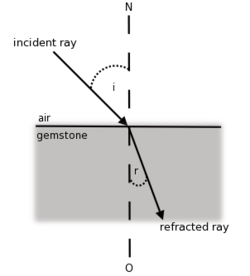# Introductory physics: refraction

Light can be refracted as well as reflected: in refraction, light is transmitted, but changes direction as it passes from one medium to another. This familiar phenomenon occurs because light has different velocities in different media. The behaviour can be explained using wave theory but luckily the main results can be described using simple geometrical optics.The tube appears bent because of the refraction of light

It can be shown that when a ray passes from one medium (1) to another (2) that is denser, the ray of light is bent towards the normal to the interface – and when a ray passes from a medium to a less optically dense one the ray is bent away from the normal. More quantitatively, the angle of incidence i (the angle between an incident ray and the normal) and the angle of refraction r (the angle between the refracted ray and the normal) are related by the simple equation

sin i/sin r = n2/n1

where n is a property of a medium known as its refractive index (related to the velocity of light in the medium)Sell’s law: sin i/sinr = n2/n1

Note the reversibility of light: if a ray bends closer to the normal upon entering water, it bends further from the normal upon leaving it i.e. all the diagrams work in either direction.

Some typical values for the index of refraction are:

 Substance n Air 1.00 Water 1.33 Glass 1.50± Plastic 1.40± Diamond 2.42

Apparent depth

One consequence of refraction is the phenomenon of apparent depth; essentially, this means that a pool of water is deeper than it appears. From the simple diagram below, you can see why (remember the observer sees the image as the intersection of the two diverging beams).The apparent depth is always shallower than the real thing, so perhaps it should be renamed apparent shallowness! It is just as well nature works this way round – if water was shallower than it appears, children would crack their heads every time they dived into a swimming pool.

Total Internal Reflection

A curious phenomenon can occur when light travels from a dense medium to a less dense one. Since a ray of light is bent away from the normal as it enters a less dense medium, it follows that at some critical angle of incidence, the refracted ray can be 90 degrees to the normal, i.e. travel along the boundary between the media. Further, rays at angles of incidence larger than the critical angle will not transmitted at all, but reflected back into the first medium. This phenomen is known as total internal reflection; the phenomenon is exploited heavily in telecommunications, where waves are transmitted undiminished over large distances in optical fibres.TIR: at large angles of incidence, the light is simply reflected back into the medium

PROBLEMS

1. If a ray of light enters water from air at an angle of incidence of 60o, calculate the angle of refraction from the table above.

2. If a person looking down vertically into a pond sees a fish apparently 18 cm below the surface, calculate the actual depth of the fish in the pond.

Filed under Introductory physics

### 3 responses to “Introductory physics: refraction”

1.Norman

A new application for Total Internal Reflection is in automatic rain detectors on modern cars. A small device behind the rear-view-mirror bounces a ray of light along the inside of the windscreen for a short distance and detects it as a totally internally reflected signal. When rain falls on the windscreen, the refractive index between the glass and the atmosphere changes, and TIR breaks down, the light escapes and the sensor detects a significant drop in the signal, thus activating the windshield wipers. Thought you might find that interesting.

2.cormac

Nice one! I didn’t know this – though I prefer to turn the wipers on whan I judge it to be necessary!

3.Freida Orandello

I can work out what to post manually, read the blog join in and so on, but what should use as the autoposted comments?